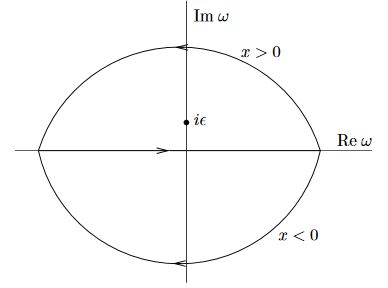Contents

# Contents

## Idea

Generally, a step function is a function from the real numbers to themselves which is constant everywhere except at one single point (or a finite number of points).

Specifically the function

$\Theta \colon x \mapsto \left\{ \array{ 0 &\vert& x \lt 0 \\ 1 &\vert& x \geq 0 } \right.$

is sometimes called the Heaviside step function.

This may be regarded as the generalized function-expression for the distributional density $\Theta \in \mathcal{D}'(\mathbb{R})$ which sends a bump function $b \in C^\infty_c(\mathbb{R})$ to its integral over the positive half-axis:

\begin{aligned} \int_{\mathbb{R}} b(x) \Theta(x) d x & \coloneqq \langle \Theta, b\rangle \\ & \coloneqq \int_0^\infty b(x) d x \end{aligned} \,.

As such $\Theta$ is also called the Heaviside distribution.

The distributional derivative of the Heaviside distribution is the Dirac delta distribution (prop. below).

## Properties

###### Proposition

The distributional derivative of the Heaviside distribution $\Theta \in \mathcal{D}'(\mathbb{R})$ is the delta distribution $\delta \in \mathcal{D}'(\mathbb{R})$:

$\partial \Theta = \delta \,.$
###### Proof

For $b \in C^\infty_c(\mathbb{R})$ any bump function we compute:

\begin{aligned} \int \partial\Theta(x) b(x) \, d x & = - \int \Theta(x) \partial b(x)\, dx \\ & = - \int_0^\infty \partial b(x) d x \\ & = - \left( b(x)\vert_{x \to \infty} - b(0) \right) \\ & = b(0) \\ & = \int \delta(x) b(x) \, dx \,. \end{aligned}
###### Definition

(Fourier integral formula for step function)

The Heaviside distribution $\Theta \in \mathcal{D}'(\mathbb{R})$ is equivalently the following limit of Fourier integrals (see at Cauchy principal value)

\begin{aligned} \Theta(x) & = \frac{1}{2\pi i} \int_{-\infty}^\infty \frac{e^{i \omega x}}{\omega - i 0^+} \\ & \coloneqq \underset{ \epsilon \to 0^+}{\lim} \frac{1}{2 \pi i} \int_{-\infty}^\infty \frac{e^{i \omega x}}{\omega - i \epsilon} d\omega \,, \end{aligned}

where the limit is taken over sequences of positive real numbers $\epsilon \in (-\infty,0)$ tending to zero.

###### Proof

We may think of the integrand $\frac{e^{i \omega x}}{\omega - i \epsilon}$ uniquely extended to a holomorphic function on the complex plane and consider computing the given real line integral for fixed $\epsilon$ as a contour integral in the complex plane.

If $x \in (0,\infty)$ is positive, then the exponent

$i \omega x = - Im(\omega) x + i Re(\omega) x$has negative real part for positive imaginary part of $\omega$. This means that the line integral equals the complex contour integral over a contour $C_+ \subset \mathbb{C}$ closing in the upper half plane. Since $i \epsilon$ has positive imaginary part by construction, this contour does encircle the pole of the integrand $\frac{e^{i \omega x}}{\omega - i \epsilon}$ at $\omega = i \epsilon$. Hence by the Cauchy integral formula in the case $x \gt 0$ one gets

\begin{aligned} \underset{\epsilon \to 0^+}{\lim} \frac{1}{2 \pi i} \int_{-\infty}^\infty \frac{e^{i \omega x}}{\omega - i \epsilon} d\omega & = \underset{\epsilon \to 0^+}{\lim} \frac{1}{2 \pi i} \oint_{C_+} \frac{e^{i \omega x}}{\omega - i \epsilon} d \omega \\ & = \underset{\epsilon \to 0^+}{\lim} \left(e^{i \omega x}\vert_{\omega = i \epsilon}\right) \\ & = \underset{\epsilon \to 0^+}{\lim} e^{- \epsilon x} \\ & = e^0 = 1 \end{aligned} \,.

Conversely, for $x \lt 0$ the real part of the integrand decays as the negative imaginary part increases, and hence in this case the given line integral equals the contour integral for a contour $C_- \subset \mathbb{C}$ closing in the lower half plane. Since the integrand has no pole in the lower half plane, in this case the Cauchy integral formula says that this integral is zero.

###### Remark

The Fourier form of the step function in prop. gives rise to the standard expression for the advanced propagator, retarded propagator and Feynman propagator used in perturbative quantum field theory. See at Feynman propagator for more.